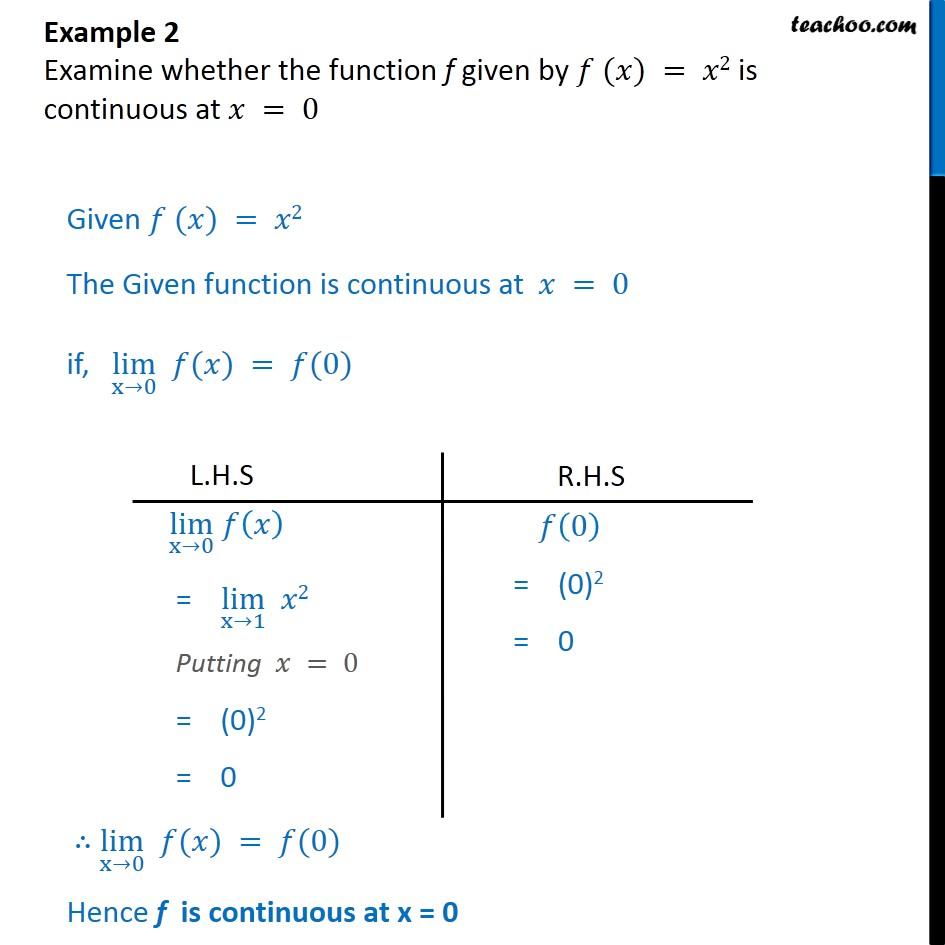Check Full Chapter Explained - Continuity and Differentiability - https://you.tube/Chapter-5-Class-12-Continuity1. Chapter 5 Class 12 Continuity and Differentiability
2. Serial order wise
3. Examples

Transcript

Example 2 Examine whether the function f given by 𝑓 (𝑥) = 𝑥2 is continuous at 𝑥 = 0 Given 𝑓 (𝑥) = 𝑥2 The Given function is continuous at 𝑥 = 0 if, ﷐lim﷮x→0﷯ 𝑓(𝑥) = 𝑓(0) ∴ ﷐lim﷮x→0﷯ 𝑓(𝑥) = 𝑓(0) Hence f is continuous at x = 0

Examples